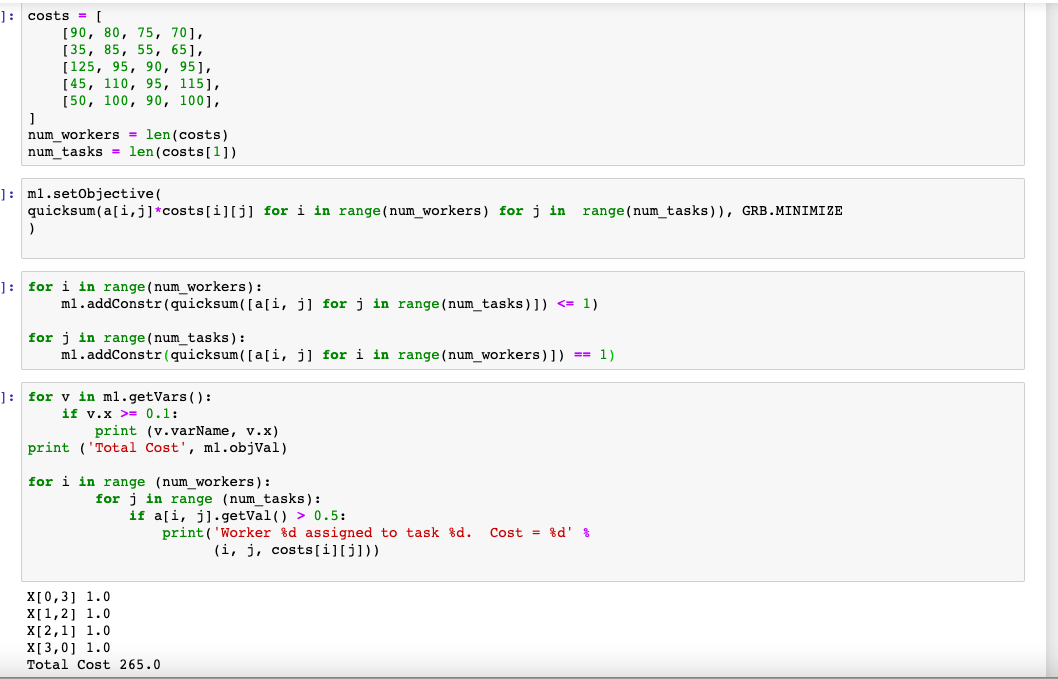•Gurobi Staff

Hi Merve,

That depends on how you are defining and computing those scores. If this is encoded in your constraints, you may want to get the corresponding constraint, get its LinExpr, and call getValue() to get the value of this constraint for the current solution, e.g.:

c = m.getConstrs()m.getRow(c).getValue()
Cheers,
Matthias
•Hi, actually matching scores (costs) are given in a matrix format as below and scores and the value of the matching is obtained by multiplying the binary variable (1 or 0) and the score as in the objective function in the code. So, it is not encoded in my constraints, so when I try the same way like  c=m.getObjective and get.Value to obtain matching scores next to each matching, it did not work. I just want to obtain something like X[0,3]  Score 75 , not X[0,3] 1.0 (binary value) as in my output.•Gurobi Staff

It seems, that a[i,j] are your variables. Why don't you just query the X attribute of those variables just like before?

for i in range(num_workers):  for j in range(num_tasks):    if a[i,j].X > 0.5:      print(f'Worker {i} assigned to task {j}. Costs = {costs[i][j]}')

Cheers,
Matthias

•Thank you very much! I could not think about it like that as I am a newbie in Python and Gurobi. Now, I obtained exactly what I wanted thanks to you!

Sincerely,

Merve.